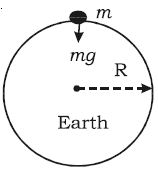Physics

# Acceleration due to Gravity at Surface of the Earth

Consider a body of mass m on the surface of the Earth as shown in the Figure. Its distance from the centre of the Earth is R (radius of the Earth).The gravitational force experienced by the body is F = GMm/R2, where M is the mass of the  Earth.

From Newton’s second law of motion. Force F = mg.

Equating the above two forces, GMm/R2 = mg

So, g = GM/R2

This equation shows that g is independent of the mass of the body rn. But, it varies with the distance from the centre of the Earth. If the Earth is assumed to be a sphere of radius R, the value of g on the surface of the Earth is given by g = GM/R2.